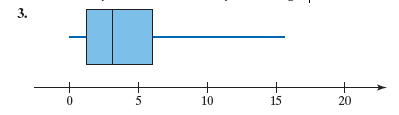×
Get Full Access to Statistics: Informed Decisions Using Data - 5 Edition - Chapter 3.5 - Problem 3
Get Full Access to Statistics: Informed Decisions Using Data - 5 Edition - Chapter 3.5 - Problem 3

×

# ?In Problems 3 and 4, (a) identify the shape of the distribution and (b) determine the five-number summary. Assume that each number in the five-numberISBN: 9780134133539 240

## Solution for problem 3 Chapter 3.5

Statistics: Informed Decisions Using Data | 5th Edition

• Textbook Solutions
• 2901 Step-by-step solutions solved by professors and subject experts
• Get 24/7 help from StudySoup virtual teaching assistantsStatistics: Informed Decisions Using Data | 5th Edition

4 5 1 241 Reviews
10
1
Problem 3

In Problems 3 and 4, (a) identify the shape of the distribution and (b) determine the five-number summary. Assume that each number in the five-number summary is an integer.Step-by-Step Solution:

Step 1 of 5) In Problems 3 and 4, (a) identify the shape of the distribution and (b) determine the five-number summary. Assume that each number in the five-number summary is an integer. In addition, the explanatory variable is not manipulated. Designed experiments are used when control of the individuals in the study is desired to isolate the effect of a certain treatment on a response variable. We introduced five sampling methods: simple random sampling, stratified sampling, systematic sampling, cluster sampling, and convenience sampling. All the sampling methods, except for convenience sampling, allow for unbiased statistical inference to be made. Convenience sampling typically leads to an unrepresentative sample and biased results.

Step 2 of 2

## Discover and learn what students are asking

Statistics: Informed Decisions Using Data : Tests for Independence and the Homogeneity of Proportions
?Profile of Smokers The following data represent the smoking status from a random sample of 1054 U.S. residents 18 years or older by level of education

Statistics: Informed Decisions Using Data : Tests for Independence and the Homogeneity of Proportions
?What’s in a Word? Part II In a recent survey conducted by the Pew Research Center, a random sample of adults 18 years of age or older living in the co

Statistics: Informed Decisions Using Data : Comparing Three or More Means (One-Way Analysis of Variance)
?The variability within each treatment group, which is a weighted average of the sample variances from each treatment where the weights are based on th

Statistics: Informed Decisions Using Data : Two-Way Analysis of Variance
?To graphically display the role interaction plays in a factorial design, we draw ______.

Statistics: Informed Decisions Using Data : Inference about the Difference between Two Medians: Dependent Samples
?Car Rentals The following data represent the weekday rental rate for a compact car charged by two car-rental companies, Avis and Hertz, in 11 location

Chemistry: The Central Science : The Chemistry of Life: Organic and Biological Chemistry
?Give the name or condensed structural formula, as appropriate: (c) 2,5,6-trimethylnonan

Unlock Textbook Solution